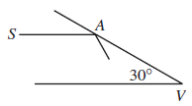# 2002COMC加拿大数学公开赛真题免费下载

### 真题与答案下载### 2002 COMC真题免费下载

Part A共8题，每题5分

Part B共4题，每题10分

#### 部分真题预览：

Part A :

6)Two mirrors meet at an angle of 30o at the point V. A beam of light, from a source S, travels parallel to one mirror and strikes the other mirror at point A, as shown. After a number of reflections, the beam comes back to S. If SA and AV are both 1 metre in length, determine the total distance travelled by the beam.7)N is a five-digit positive integer. A six-digit integer P is constructed by placing a 1 at the right-hand end of N. A second six-digit integer Q is constructed by placing a 1 at the left-hand end of N. If P is three times Q, determine the value of N.

8)Suppose that M is an integer with the property that if x is randomly chosen from the set { 1,2,3,...,999,1000}, the probability that x is a divisor of M is 1/100 . If M ≤ 1000, determine the maximum possible value of M.

Part B :

1)Square ABCD has vertices A(0,0), B(0,8), C(8,8), and D(8,0). The points P(0,5) and Q(0,3) are on side AB , and the point F(8,1) is on side CD.

1. What is the equation of the line through Q parallel to the line through P and F?
2. If the line from part (a) intersects AD at the point G, what is the equation of the line through F and G?
3. The centre of the square is the point H(4,4). Determine the equation of the line through H perpendicular to FG.
4. A circle is drawn with centre H that is tangent to the four sides of the square. Does this circle intersect the line through F and G? Justify your answer. (A sketch is not sufficient justification.)

### 2002 COMC加拿大数学奥赛完整版答案免费下载